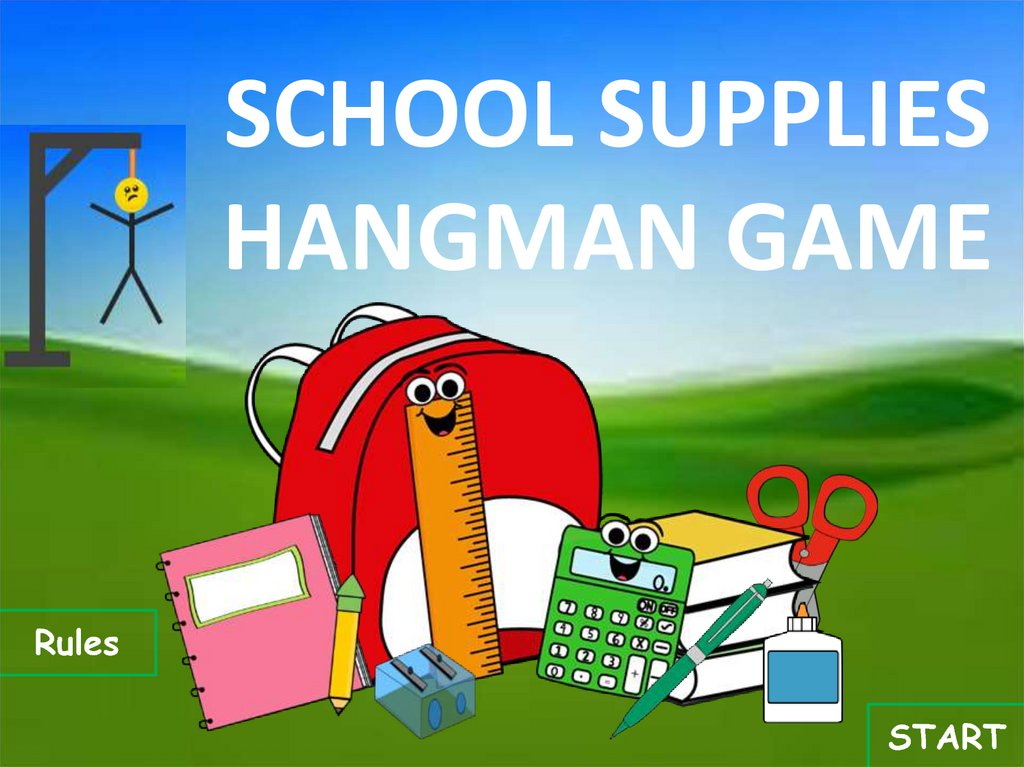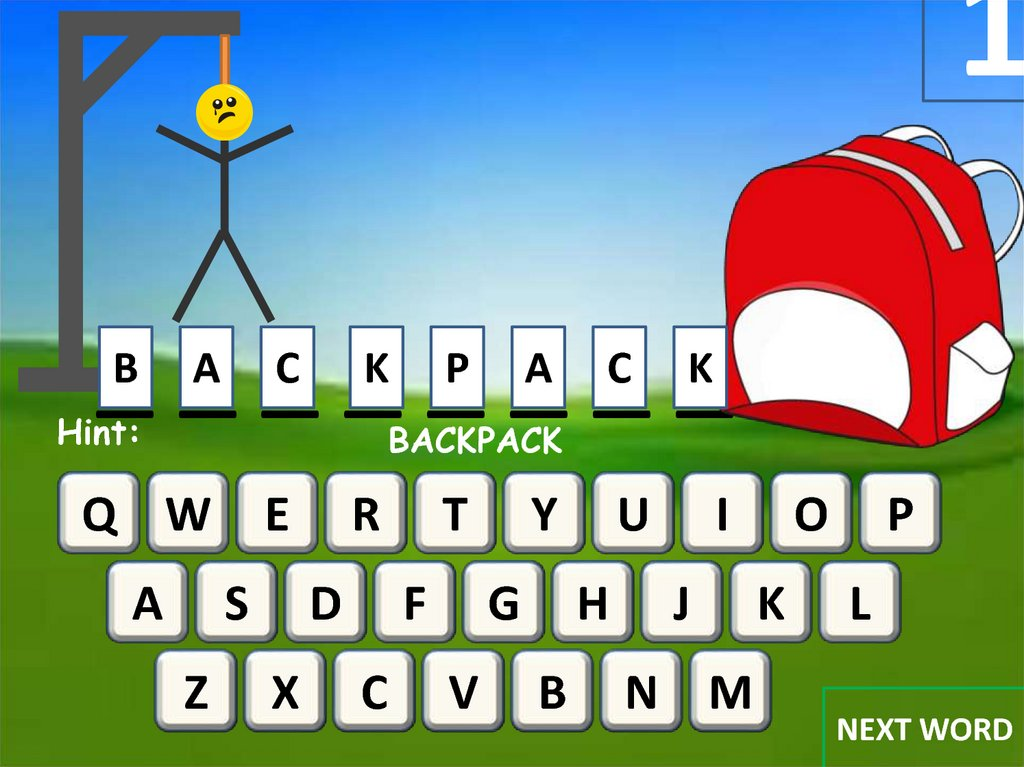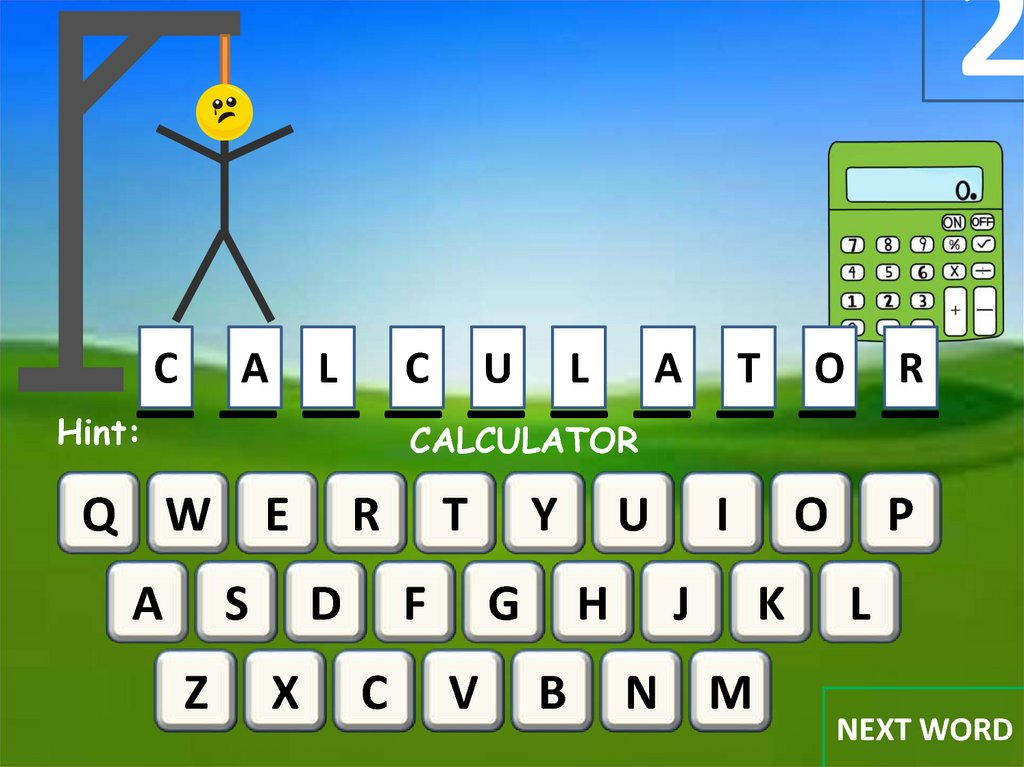School supplies

SCHOOL SUPPLIES
HANGMAN GAME
Rules
START

2. 2. dia

1
_B _A _C _K _P _A _C _K
Hint:
BACKPACK
Q W
A
E
S
Z
R
D
X
T
F
C
Y
G
V
U
H
B
I
J
N M
O
K
P
L
NEXT WORD

3. 3. dia

2
_C _A _L _C _U _L _A _T _O _R
Hint:
CALCULATOR
Q W
A
E
S
Z
R
D
X
T
F
C
Y
G
V
U
H
B
I
J
N M
O
K
P
L
NEXT WORD

4. 4. dia

3
PENCIL SHARPENER
Hint:
_P _E _N _C _I _L
_S _H _A _R _P _E _N _E _R
Q W
A
E
S
Z
R
D
X
T
F
C
Y
G
V
U
H
B
I
J
N M
O
K
P
L
NEXT WORD

4
_R _U _L _E _R
Hint:
RULER
Q W
A
E
S
Z
R
D
X
T
F
C
Y
G
V
U
H
B
I
J
N M
O
K
P
L
NEXT WORD

5
_G _L _U _E
Hint:
GLUE
Q W
A
E
S
Z
R
D
X
T
F
C
Y
G
V
U
H
B
I
J
N M
O
K
P
L
NEXT WORD

7. 7. dia

6
_ O_ T_ E_ _B O_ O_ K_
N
Hint:
NOTEBOOK
Q W
A
E
S
Z
R
D
X
T
F
C
Y
G
V
U
H
B
I
J
N M
O
K
P
L
NEXT WORD

8. 8. dia

7
_P _E _N _C _I _L
Hint
PENCIL
Q W
A
E
S
Z
R
D
X
T
F
C
Y
G
V
U
H
B
I
J
N M
O
K
P
L
NEXT WORD

8
BOOKS
_B _O _O _K _S
Hint:
Q W
A
E
S
Z
R
D
X
T
F
C
Y
G
V
U
H
B
I
J
N M
O
K
P
L
NEXT WORD

10. 10. dia

9
Hint:
_S _C _I _S _S _O _R _S
SCISSORS
Q W
A
E
S
Z
R
D
X
T
F
C
Y
G
V
U
H
B
I
J
N M
O
K
P
L
NEXT WORD

10
_P _E _N
Hint:
PEN
Q W
A
E
S
Z
R
D
X
T
F
C
Y
G
V
U
H
B
I
J
N M
O
K
P
L

12. 12. dia

Rules
Instructions for Playing Hangman
Have the students select a letter of the alphabet.
Click on the keyboard button with the appropriate letter on it.
If the letter is contained in the word/phrase, it will appear on
the lines.
If the letter is not contained in the word/phrase, a portion of